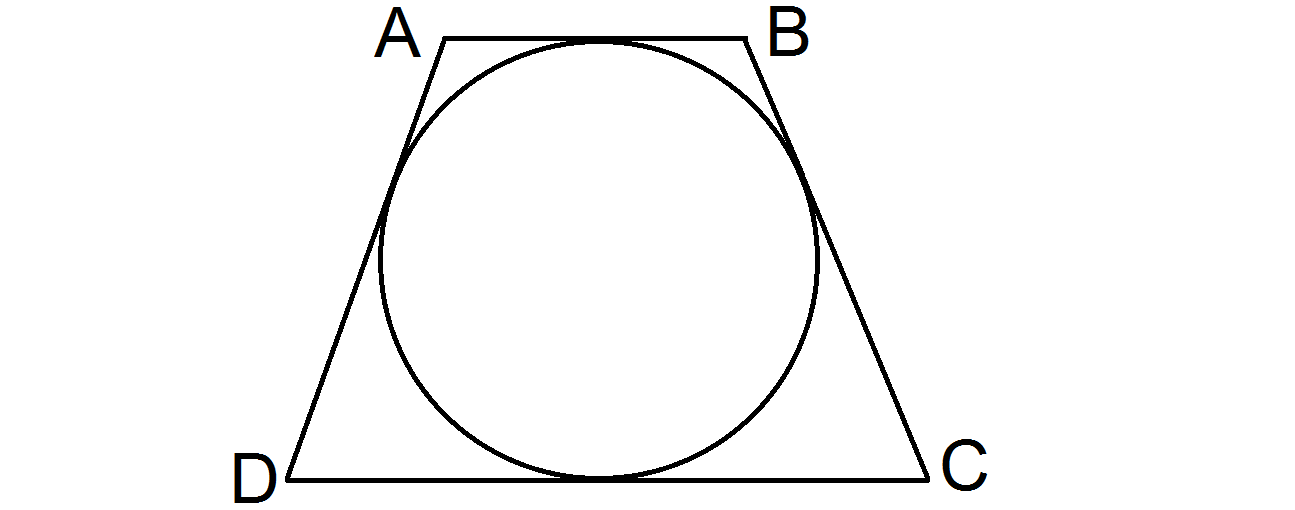# Tangential Trapezoid$ABCD$ is a tangential isosceles trapezoid, touching the circle as shown above.

If the perimeter of the trapezoid is 52, and the circle's radius and all trapezoid's sides have lengths in whole integers, what is the area of the trapezoid?

×